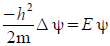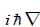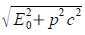# Why did Nature Invent Spin?I think this issue receives too little attention. Usually, it is said that spin is a consequence of the Dirac equation and thus, something that follows necessarily from relativity and quantum mechanics. Let us have a brief look at the argument. Schrödinger’s non-relativistic equation is.

The momentum operator p = m v isand thus, the term on the left-hand side of Schrödinger’s equation is derived simply from the kinetic energy  ½ mv2. It is interesting that none of the successful predictions of Schrödinger’s equation for the hydrogen atom make specific reference to the nature of the electron (for which the wave function gives a probability that it will be found in a certain state). They refer only to the kinetic energy; irrespective of the type of wave-natured particle that orbits the nucleus (in fact, it also works for muonic atoms).

Dirac used the correct special relativistic term for energy E =and replaced Schrödinger’s term. However, there was no explicit justification for switching from the kinetic energy to the total energy of the particle. This conceptual problem was overshadowed somehow by the mathematical problem arising from the Delta operator in the square root, to which Dirac found an ingenious solution using the matrices named after him. The algebra of Dirac matrices transpired to be a description of spin. In the following, the opinion spread that spin was a consequence of putting relativistic energy into the basic equations of quantum mechanics.

However, the initial problem of the missing equivalence of kinetic and total energy persisted. Dirac was also disappointed that he could not deduce any concrete properties for the electron from his equation. The retrospective narrative is that the positron, undiscovered in 1928, was a ‘prediction’ of Dirac’s theory, but Dirac had rather sought to explain the huge mass relation of the proton and electron, which is 1836.15. In fact, in his latter days, Dirac distanced himself a little from his earlier findings and according to his biographer Helge Kragh, he was “disposed to give up everything for what he had become famous”.

Let us adopt another perspective regarding the nature of spin, one which is related to the properties of three-dimensional space; the world we perceive (those who perceive more dimensions should see a doctor). The group of rotations SO(3) obviously must have some significance, but its topology is a little intricate. It lacks a property called ‘simple connectedness’ because the paths in SO(3) may not be contracted. Objects connected to a fixed point with a ribbon must twist 720 degrees, not just 360 degrees, in order to perform a full rotation that leaves the ribbon untwisted (see the visualization here). It seems that nature has a predilection for the generalized rotations called SU(2), which are simpler mathematically and have a surprising feature; they represent precisely the electron’s spin – you need to perform a double twist of 720 degrees, rather than just 360 degrees, to get into the original position. However, there are no Dirac matrices and thus, I think there is an open problem. It seems that the properties of three-dimensional space alone are sufficient to cause spin to emerge – no relativity or quantum mechanics are needed. To put it another way, a direct understanding of quantum mechanics from the geometrical properties of space, if there is one, is still missing.